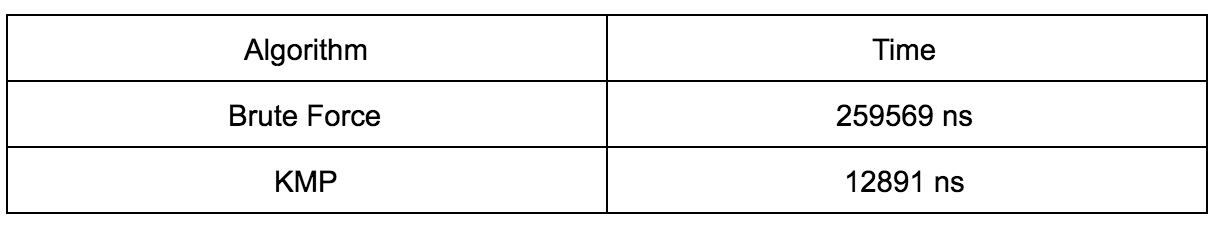# Introduction

We have seen brute force approach to string search here. Brute force approach to string search has time complexity of O(n*m). Donald Knuth and Vaughan Pratt, and James H. Morris conceived the algorithm in 1970. KMP algorithm is the first algorithm to have linear time complexity.

# Problem Statement

We will assume text (where we have to search) is an array of n characters. Let’s denote the text by T[1..n]. String to be searched is called pattern and is represented by an array P[1..m] where m ≤ n.

We need to find indexes in given text T where the given pattern P occurs. We need to improve from the brute force algorithm having time complexity of O(m*n).

Refer here for detailed problem statement.

# Explanation of KMP Algorithm

Here’s our text and pattern:

We will try matching from first character of pattern with first character of the text. Both are “a” and it matches. Moving on, we match second character which is “b” and it matches. But the third character also matches which is “a”. Fourth character is “b” in text but “c” in pattern and they do not match. Following picture illustrate this.

The matched substring is “aba” in pattern “abac” from index 0 to 2 in text as well as pattern.

If we observe closely, the matched substring has “a” in prefix which is also suffix in the same substring. Following figure illustrates this.

Now, using the fact that we have already transversed the string, we will not start matching pattern from index 0 with text as index 1. Instead, we will match text at index 3 and pattern at index 1. Because, we know that character at index 2 in text, i.e. “a”, is already present in pattern at index 0. This is the crux of KMP. Instead of transversing the pattern for every character in text, we transverse the pattern once and find the information about repetition.

We again continue matching until we find mismatch at index 4 of text with index 2 of pattern. Following picture illustrates this.

The matched substring is ab. We don’t have any prefix and suffix that matches. In this case, we will start matching again with text at index 3 and pattern at index 0.

Continue transversing.

# Implementation

We first transverse the pattern and find information about repetition.

We will transverse the pattern character array and try to build suffix array. Suffix array will have length equal to pattern character array. First position of suffix will be always 0 as there is no index before 0th index of pattern character array. Or in other words, there is no character “a”, before the first “a” in pattern. See figure 7 for reference.

Our suffix array will look like this after each iteration:So, only “a” at index 2 is already present at index 0, so suffix array has 1 at second index.

```Question: What would be the suffix array for pattern "abab"?

## Code

This file contains bidirectional Unicode text that may be interpreted or compiled differently than what appears below. To review, open the file in an editor that reveals hidden Unicode characters. Learn more about bidirectional Unicode characters
 import java.util.ArrayList; import java.util.List; public class KMP { public static final int NOT_FOUND_INDEX = –1; public static void main(String args[]){ System.out.println("**************** Knuth-Morris-Pratt *****************"); String text = "abcabac"; String pattern = "abac"; long initialTime = System.nanoTime(); int index = findPatternInText(pattern, text); long finalTime = System.nanoTime(); if(index == NOT_FOUND_INDEX) System.out.println("Pattern is not find in the text"); else System.out.println("Successful! Pattern found at index "+index); System.out.println("Time taken for matching "+(finalTime – initialTime)); } private static int findPatternInText(String pattern, String text) { int index = NOT_FOUND_INDEX; int patternLength = pattern.length(); int textLength = text.length(); char[] textCharArray = text.toCharArray(); char[] patternArray = pattern.toCharArray(); int[] suffixArray = generateSuffixArray(patternLength, pattern); int i = 0; boolean isMatched = true; int j = 0; while(j < patternLength && i < textLength){ if(textCharArray[i] == patternArray[j]){ j = j + 1; i =i + 1; } else{ int newIndex = i – j + 1; isMatched = false; j = j – 1; if (j < 0) j = 0; j = suffixArray[j]; if(j==0) i =newIndex; else i = i + 1; } if(j == patternLength) { isMatched = true; } } if(isMatched) index = i – patternLength; return index; } private static int[] generateSuffixArray(int patternLength, String pattern) { int[] suffixArray = new int[patternLength]; char[] patternArray = pattern.toCharArray(); int i = 1; while(i < patternLength){ for(int j = 0; j < patternLength; j++){ if((i < patternLength) && (patternArray[i] == patternArray[j])){ suffixArray[i] = j + 1; i = i + 1; } else{ i = i + 1; break; } if(i == patternLength – 1) break; } } return suffixArray; } }
view raw KMP.java hosted with ❤ by GitHub

# Time Complexity

The time complexity of suffix generation is O(m) where m is the length of pattern. Time complexity of finding pattern is O(n) where n is the length of the pattern. Total time complexity is O(m+n).

# Time ComparisonWhen text = abcabac” and pattern = “abac”.

Reference:

1

2

3

4

If you liked this article and would like one such blog to land in your inbox every week, consider subscribing to our newsletter: https://skillcaptain.substack.com

## 2 thoughts on “Simple Explanation And Implementation Of Knuth-Morris-Pratt (KMP) Algorithm For String Search”

1.Sha3 says: